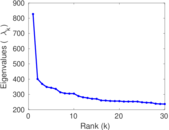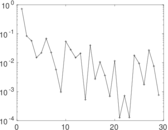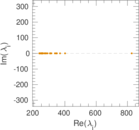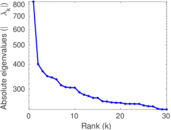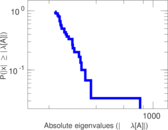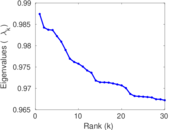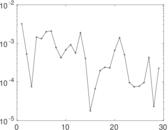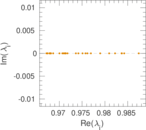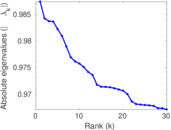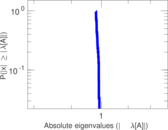This network consists of the wikilinks of the Wikipedia in the Dutch language (nl). Nodes are Wikipedia articles, and directed edges are wikilinks, i.e., hyperlinks within one wiki. In the wiki source, these are indicated with [[double brackets]]. Only pages in the article namespace are included.

 Code `Wnl` Internal name `wikipedia_link_nl` Name Wikipedia links (nl) Data source http://dumps.wikimedia.org/ AvailabilityDataset is available for download Consistency checkDataset passed all tests Category Hyperlink network Node meaning Article Edge meaning Wikilink Network format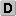Unipartite, directed Edge typeUnweighted, no multiple edges ReciprocalContains reciprocal edges Directed cyclesContains directed cycles Loops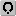Contains loops

## Statistics

 Size n = 2,622,756 Volume m = 59,168,169 Loop count l = 2,545 Wedge count s = 4,173,770,833,329 Claw count z = 1,133,281,298,331,688,960 Cross count x = 2.434 52 × 1023 Triangle count t = 816,051,566 Maximum degree dmax = 900,368 Maximum outdegree d+max = 10,515 Maximum indegree d−max = 900,336 Average degree d = 45.119 1 Size of LCC N = 2,622,230 Diameter δ = 10 50-Percentile effective diameter δ0.5 = 3.106 52 90-Percentile effective diameter δ0.9 = 4.314 96 Median distance δM = 4 Mean distance δm = 3.517 45 Balanced inequality ratio P = 0.197 093 Outdegree balanced inequality ratio P+ = 0.268 062 Indegree balanced inequality ratio P− = 0.135 162 Degree assortativity ρ = −0.120 627 Degree assortativity p-value pρ = 0.000 00 Clustering coefficient c = 0.000 586 557 Directed clustering coefficient c± = 0.409 479 Operator 2-norm ν = 3,000.53 Cyclic eigenvalue π = 827.192 Spectral separation |λ1[A] / λ2[A]| = 1.007 09 Reciprocity y = 0.395 464 Non-bipartivity bA = 0.006 990 40 Normalized non-bipartivity bN = 0.027 370 9

## Plots

### Degree distribution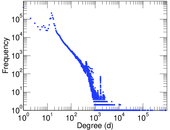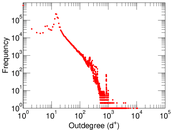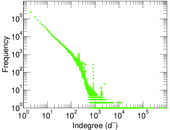### Cumulative degree distribution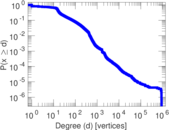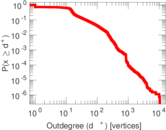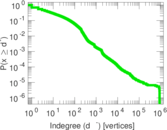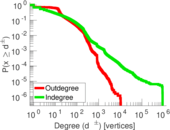### Zipf plot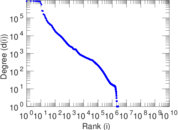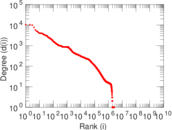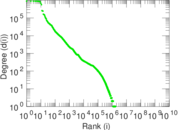### Hop distribution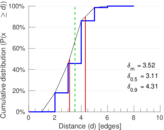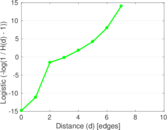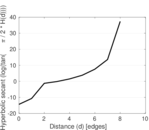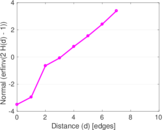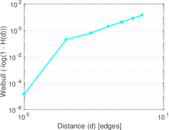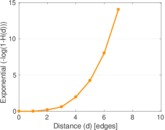### In/outdegree scatter plot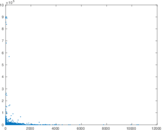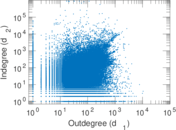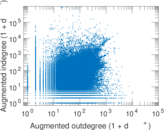### Matrix decompositions plots